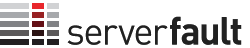0

(click on this box to dismiss)Q&A for system and network administrators

SELECT
COUNT(DISTINCT p.id) as posts,
(SELECT
COUNT(DISTINCT v.postid)
left join posts p
on p.id = v.postid
where v.votetypeid = '3'
and p.creationdate > '2014-06-01')
(SELECT COUNT(v.id)
left join posts p
on p.id = v.postid
where v.VoteTypeId = '3'
and p.creationdate > '2014-06-01'
(SELECT
COUNT(DISTINCT v.postid)
left join posts p
on p.id = v.postid
where v.votetypeid = '2'
and p.creationdate > '2014-06-01')
(SELECT COUNT(v.id)
left join posts p
on p.id = v.postid
where v.VoteTypeId = '2'
and p.creationdate > '2014-06-01'
COUNT(c.id)/COUNT(DISTINCT p.id) as ComPerPost,
COUNT(CASE when v.votetypeid in ('4','6','10','12','15') then v.id end) as votesDel,
CAST(
CAST(COUNT(CASE when v.VoteTypeId = '2' then v.id end) as DECIMAL(20,5))
/
CAST(COUNT(DISTINCT p.id) as DECIMAL(20,5))
as DECIMAL(20,5)) as voteweightUp,
CAST(
CAST(COUNT(CASE when v.VoteTypeId = '3' then v.id end) as DECIMAL(20,5))
/
CAST(COUNT(DISTINCT p.id) as DECIMAL(20,5))
as DECIMAL(20,5)) as voteweightDown
FROM posts pHold tight while we fetch your results